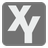Reverse Engineer
Challenging
English

You are standing next to a math machine which takes two numerical inputs x and y, and has a single numerical output. It is known that the machine evaluates an expression using x, y, the operators + – * / and brackets. You should recover the expression by using the machine and the inputs of your choice over several turns. x, y and the result you receive are integers. The machine uses real division(/) in the expression. When dividing by zero, the result will evaluate to "ZeroDivisionError".

Equivalent expressions will be accepted by the grader. For this the machine evaluates your expression and the hidden expression several times using random values.

In each step your function gets a list with data from the past steps. Each element is a list of three numbers -- x(int), y(int) and output(a fraction as a list or the string "ZeroDivisionError"). The output is represented as a list with two integers - numerator and denominator.

The function should return a list with three elements -- your guess as an expression string, x and y, as integers.

Input: A list with data from the past steps.

Output: A list with three elements -- your guess as an expression string, x (int) and y (int).

Example:

```checkio([])  # hidden expression "x+y"
checkio([[2, 2, [4, 1]]]) # ["x*y", 3, 3]
checkio([[2, 2, [4, 1]], [3, 3, [6, 1]]])  # ["x+y", 5, 5]```

How it is used: The concepts used in this task can help you to understand someone else’s code and add new techniques to your own repertoire. In addition to this, reverse engineering comes in handy in cryptology where it can be used to decrypt coded information.

Precondition: The hidden expression is correct. It does not evaluate at ZeroDivisionError always.